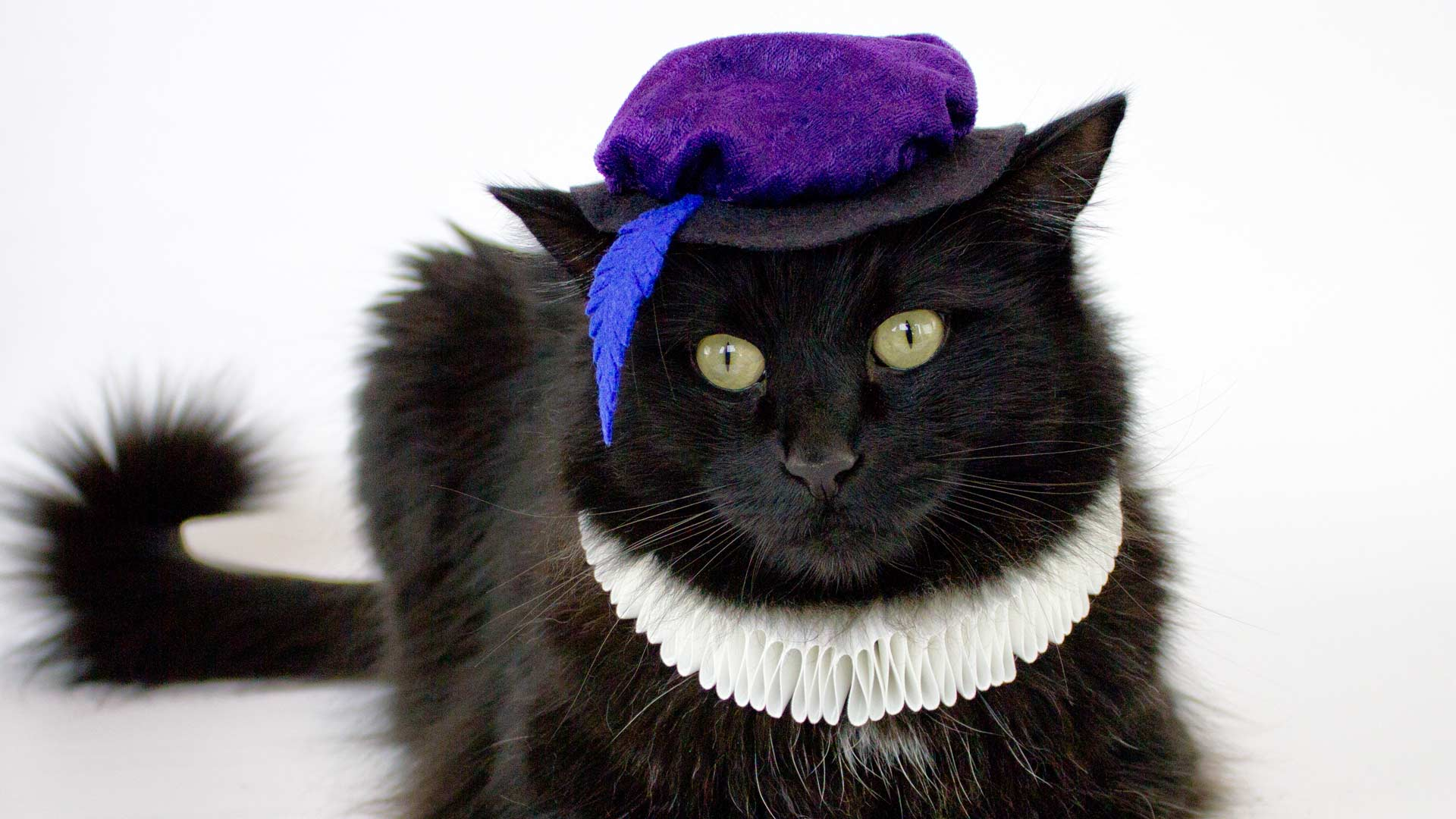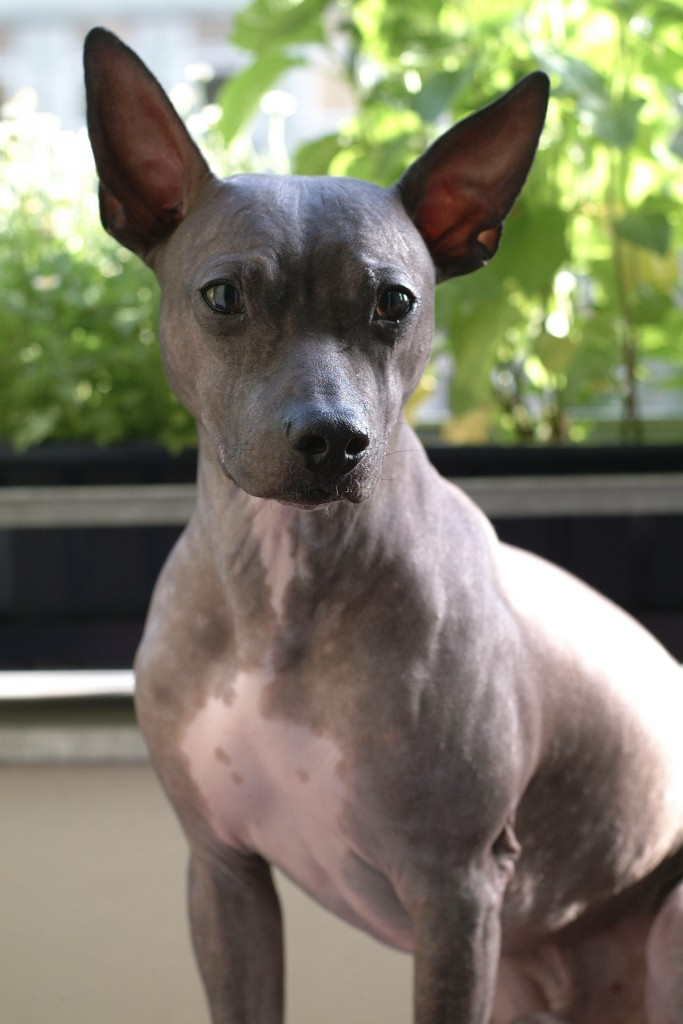### Convolutional Neural Network for Cat vs Dog Image Classification

The goal of this project was to experiment with a basic convolutional neural network whose purpose is to distinguish whether an inputted image is that of a cat or dog. This simple network is an example of the powerful capabilities of machines to learn and recognize what objects are. This network can easily be adapted to distinguish between more animals or completely different objects with some modifications and corresponding data sets.

Convolution Stages:

The convolution stage of this CNN begins with a 128x128 RGB image. All the convolutions are followed by batch normalization, then by a rectifier activation function, and finally by a 2x2 max pooling kernel. All the convolution filters are of size 3x3 with a stride of 1. The kernel initializer used was the default Xavier normal initializer. The ReLU activation function is used to eliminate (set the value to zero) the pixels where the convolution kernel does not detect any features. Batch normalization is used after each convolution layer to make sure the data stays normalized as its passed through the layers. The first layer uses 8 filters, followed by 16, then 32, and finally 64. With max pooling after each stage, we end with an image of size 8x8.

Hidden Layer:

This network only has one hidden layer with a size of 512 neurons. The input must be a vector so all the feature maps are flattened to a single vector before being fed into the hidden layer. Using 512 neurons gives the advantage of being able to detect more complicated patterns. However, with a network that only distinguishes between cats and dogs, it’s important to not let the network get lost in the details and not be able to generalize well. To do this, a Dropout layer is added which drops 50% of the neurons while learning to encourage higher quality neurons to have more weight. The ReLU activation function is also used in the hidden layer.

Output Layer:

The final node of this CNN, is a single output using the sigmoid activation function. This activation function will give the output a number from 0 to 1. A 1 would mean the network has 100% confidence in the image being a dog, and 0 would be 100% confidence in the image being a cat.

Results:All training was done with a batch size of 32 using 25 epochs with 8000 training steps per epoch and 2000 validation steps. Overall this network achieved a maximum test accuracy of 91%. By bumping up the input size to 256x256, the accuracy was improved to 93.55% however training time was greatly increased.

Challenging the Network:

In order to the find the limits of this network, I needed to find some weird pictures of cats and dogs. My stargety was to find pictures of the animals wearing or holding items, stretching or scratching themselves, and animals with abnormal fur such as puffy or missing fur.

Objects:After some testing the network did very well with the animals holding or wearing objects. As long as the costume or object is not physically concealing the animal, the network had no problems.

Stretching/Scratching:This seems to be the acheleus heel of the network. Pictures of dogs with thick coats who are scratching themselves proved to be challenging for the network. Futhermore, pictures of cats stretching almost always resulted in the network thinking the animal is a cat.

Abnormal Fur:Dogs were no fur seem almost always get classified as a cat, however furless cats get classified as cats. There were to be a strong bias towards cat when there is no fur. Furthermore, puppies who have poofy coats also tend to get miscategorized as cats.

Code:

import os
import importlib
from keras import backend;
from keras import backend as K
def set_keras_backend(backend):
if (backend == "theano"):
os.environ['THEANO_FLAGS'] = "device=cuda0,force_device=True,floatX=float32"
os.environ['KERAS_BACKEND'] = backend
assert K.backend() == backend
if (backend == "tensorflow"):
assert K.backend() == backend
set_keras_backend("tensorflow")

from keras.models import Sequential
from keras.layers import Conv2D
from keras.layers import MaxPooling2D
from keras.layers import Flatten
from keras.layers import Dense
from keras.layers import Dropout
from keras.layers import Activation
from keras.layers.normalization import BatchNormalization
from keras import regularizers
from keras.preprocessing.image import ImageDataGenerator

classifier = Sequential()

# Convolution
classifier.add(Conv2D(8, (3, 3), input_shape = (128, 128, 3)))

classifier.add(Conv2D(16, (3, 3), input_shape = (128, 128, 3)))

classifier.add(Conv2D(32, (3, 3), input_shape = (128, 128, 3)))

classifier.add(Conv2D(64, (3, 3), activation = 'relu'))

# Flatten

# Full connection
classifier.add(Dense(units = 512, kernel_regularizer = regularizers.l2(0.01)))
classifier.add(Dense(units = 1, activation = 'sigmoid'))

classifier.compile(optimizer = 'adam', loss = 'binary_crossentropy', metrics = ['accuracy'])

#Training
#Creating more images by rescaling, zooming, flipping, roating original pictures
train_datagen = ImageDataGenerator(rescale = 1./255,
shear_range = 0.2,
zoom_range = 0.2,
horizontal_flip = True)

test_datagen = ImageDataGenerator(rescale = 1./255)

training_set = train_datagen.flow_from_directory('dataset/training_set',
target_size = (128, 128),
batch_size = 32,
class_mode = 'binary')

test_set = test_datagen.flow_from_directory('dataset/test_set',
target_size = (128, 128),
batch_size = 32,
class_mode = 'binary')

classifier.fit_generator(training_set,
steps_per_epoch = 8000,
epochs = 25,
validation_data = test_set,
validation_steps = 2000, verbose = 1)

#Making predictions
import numpy as np
from keras.preprocessing import image
test_image = image.load_img('dataset/single_prediction/cat_or_dog_23.jpg', target_size = (256, 256))
test_image = image.img_to_array(test_image)
test_image = np.expand_dims(test_image, axis = 0)
result = classifier.predict(test_image, verbose=1)

if (round(result) == 1):
prediction = 'dog'
confidence = 100*result
else:
prediction = 'cat'
confidence = 100 - 100*result
plot_model(classifier, to_file='model.png', show_layer_names=True, show_shapes=True, rankdir='LR' )